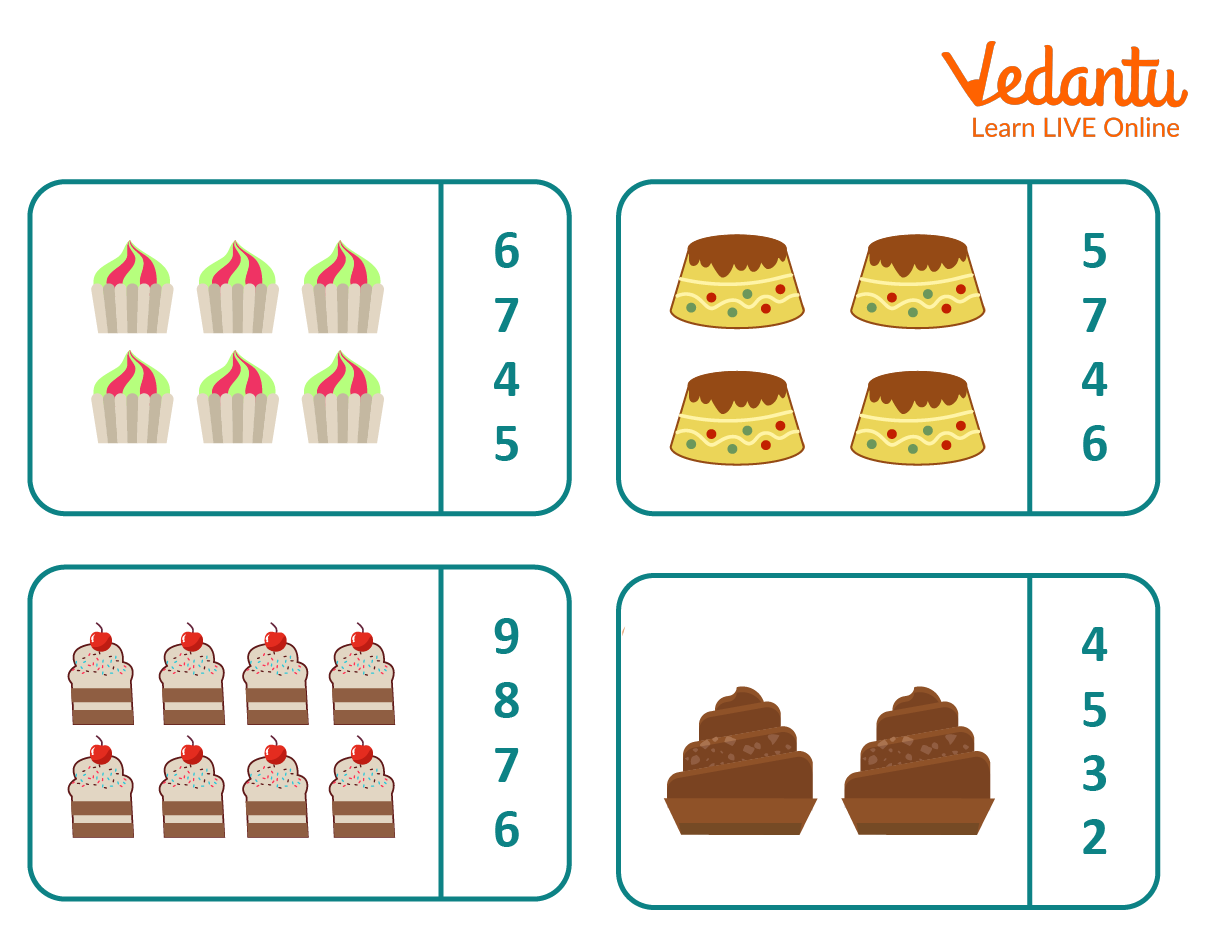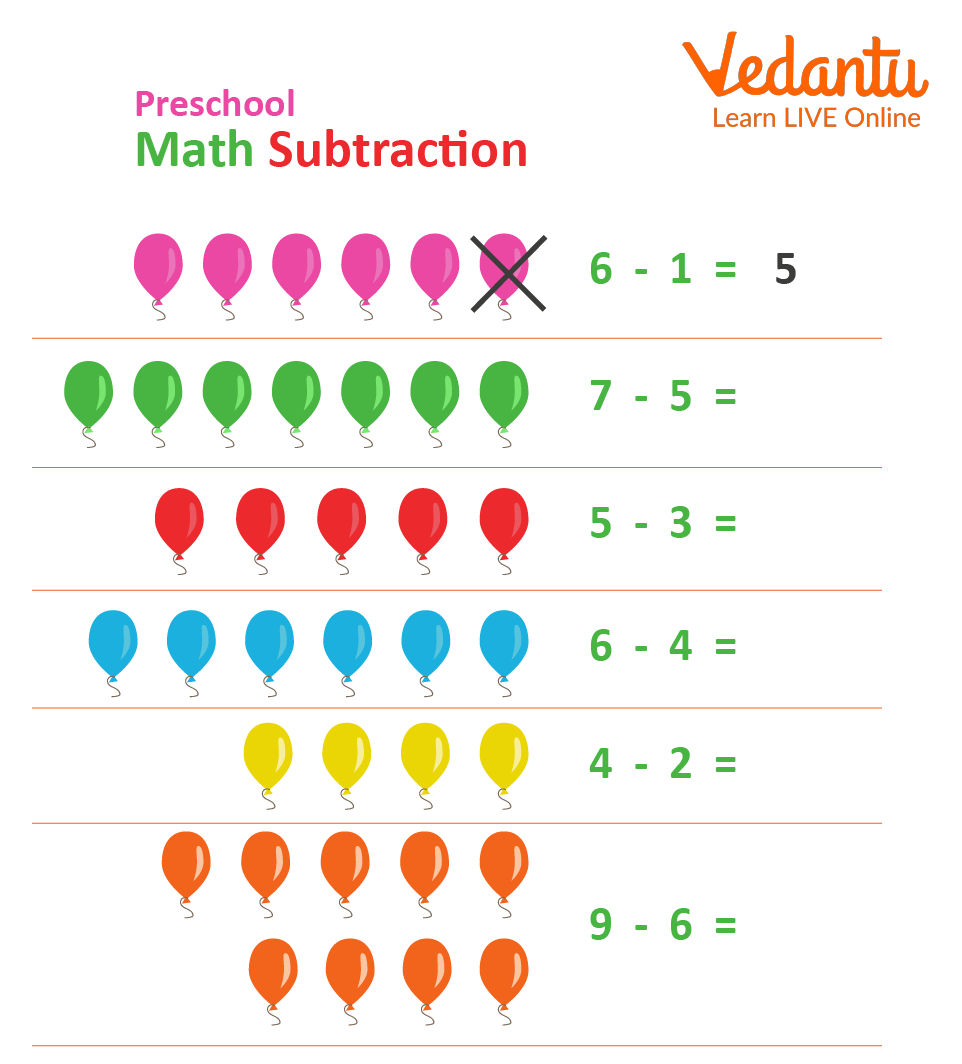Courses
Courses for Kids
Free study material
Offline Centres
More

# How to Teach Maths to Nursery Kids of Age 3-4 years?Last updated date: 03rd Dec 2023
Total views: 157.8k
Views today: 1.57kMaths is an interesting subject. The first step is to understand numbers. For example, you write two numbers 3 and 4 on the blackboard and ask your kids to add these numbers. Here, the kids as beginners firstly learn to write 3 and 4 on the paper with your aid. Now, you help them with counting the steps to take after ‘3’ to get the answer ‘7’.

Understanding numbers and mathematical operations are new for young kids. So, we follow a fundamental method to teach nursery kids.

## Steps to Teach Maths to Nursery Kids

Teaching Maths begins with learning numbers. You can help kids learn to count the numbers with various strategies that we will be discussing now. Below are the best 4 strategies to teach nursery Maths to kids of age 3-4 years.

Kids can memorise numbers faster when they develop a habit of counting or picking objects from one to ten. Once your kid begins counting, you can start with some basic Maths operations like addition and subtraction.

• Use Everyday Objects

You can use everyday objects to teach Maths to your child. For example, you can ask your kid to count the number of books, fruits, trees, or cars. You can count a few objects first, and ask your kid to count the remaining objects. Maths is easy to teach when you provide your child with real-life objects. It leaves an everlasting impression on the child.

So, counting various real-life objects instead of reading out the numbers 1, 2, 3, and so on helps your child understand numbers better.

• Play Maths Games

There are various Maths games that you can make your child play offline as well online. For example, you throw 5 balls on the floor and ask your child to count those. The next time, you may throw six balls one by one and ask your child to count them as you throw. These physical activities motivate kids to become a pro at counting numbers.

• Pattern in Maths

You can write numbers like 3, 4, __, __, and ask your child to fill the next two blanks, and practising similar series and patterns will help your kid remember the numbers better.

Now, let us have a look at the nursery Maths worksheet to understand how you can teach Maths to your kids through sample questions.

## Nursery Maths Worksheet

Worksheet 1:

In the below worksheet, you need to find the number of items in each of the four boxes, respectively. Choose the correct answer.Counting number nursery maths worksheet

The number of cupcakes in the first box:

1. 6

2. 7

3. 4

4. 5

The number of items is 6. So, option a is the correct answer.

The number of cakes in the secound box:

1. 5

2. 7

3. 4

4. 6

The number of items is 4. So, option c is the correct answer.

The number of pastries in the third box:

1. 9

2. 8

3. 7

4. 6

The number of items is 8. So, option b is the correct answer.

The number of items in the fourth box is:

1. 4

2. 5

3. 3

4. 2

The number of items is 2. So, option d is the correct answer.

Worksheet 2Subtracting numbers nursery Maths worksheet

First: 6 - 1 = 5 (given). Now, let us find the solution for the other questions.

Second: 7 - 5 = 2

Third: 5 - 3 = 2

Fourth: 6 - 4 = 2

Fifth: 4 - 2 = 2

Sixth: 9 - 6 = 3

So, this is how we can teach Maths to nursery kids with the help of real-life objects, playing Maths games, and solving worksheets. Practice makes a man perfect, so practice can help your kid become a pro in Mathematics at an early age.

## FAQs on How to Teach Maths to Nursery Kids of Age 3-4 years?

1. How to teach Maths to kids in preschool?

• Play With Shapes: Make shape pictures and ask your kid to name them. Also, ask them to count the number of sides of each shape.

• Puzzles - Make a series of numbers and ask kids to write the next digit.

2. What are the basic Mathematics skills?

The basic Maths skills for preschoolers are as follows:

• Number sense

Number sense is the ability to understand numbers and the relationships between numbers.

• Learning numbers via representation or pictures

Showing real-life objects like pens, paper, books, and so on helps children relate to mathematical concepts easily.

• Addition and subtraction of numbers

Providing questions on addition and subtraction is the best mental exercise for kids which when brought into habit works as the most helpful resource ever.